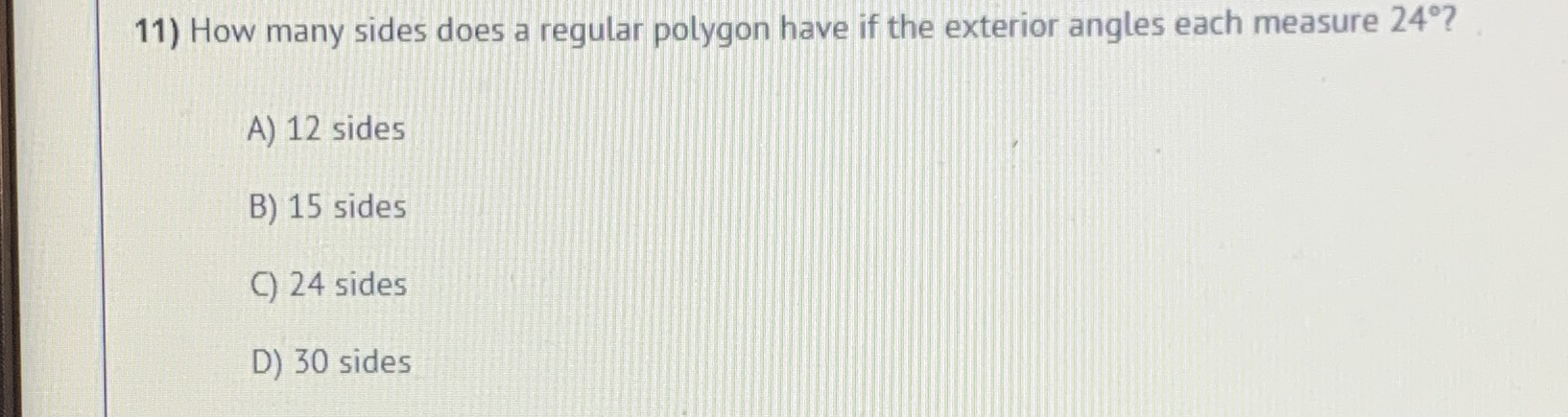### Still have math questions?

Arithmetic
Question11) How many sides does a regular polygon have if the exterior angles each measure $$24 ^ { \circ } ?$$

A) $$12$$ sides B) $$15$$ sides C) $$24$$ sides D) $$30$$ sides